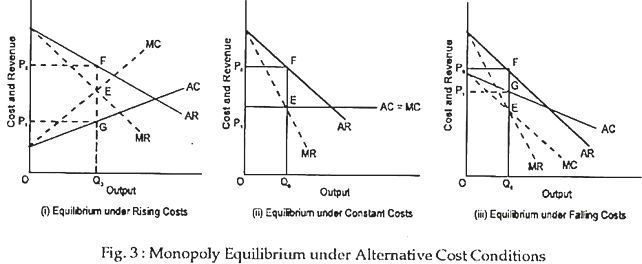# Equilibrium of the Monopolist | Markets | Economics

Equilibrium of the Monopolist under Alternative Cost Conditions !

An important difference between perfect competition and monop­oly may now be noted. While under perfect competition it is possible for a firm to reach equilibrium only when marginal cost is rising at the point of equilibrium, monopoly equilibrium may be reached under alternative cost conditions. In other words, a monopoly firm can reach equilibrium whether MC is falling, remaining constant or rising at the equilibrium level of output.

In fact, the sufficient condition of profit-maximisation (i.e., the MC curve has to intersect the MR curve from below and not from above) can be satisfied in monopoly under alternative cost conditions (i.e., in all the three cases mentioned above). But in perfect competition the sufficient condition can be satisfied only when the MC curve is rising.

“Equilibrium for the firm under perfect competition can only occur when the marginal cost of the firm is rising at or near equilibrium output.” Equilibrium under monopoly can occur whenever marginal costs are rising, falling a constant. This point may now be explained. In perfect competition the MR curve is a horizontal straight line.

So it is quite obvious that the MC curve can intersect the MR curve from below only when the MC curve is rising. In contrast, the MR curve slopes downward from left to right in monopoly. Therefore, the MC curve can intersect the (downward sloping) MR curve from below whether the MC curve is falling, remaining constant or rising.The equilibrium of a monopoly firm under alternative cost conditions is illustrated in Fig. 3. In Fig. 3(a), we observe that the MC is falling. So the MC curve slopes downward from left to right. In Fig. 3(b) MC is constant, end so that MC curve is a straight line. In Fig. 3(c) MC is rising. So the MC curve slopes upward from left to right. In each case equilibrium occurs at point £ and equilibrium output is Q0 and equilibrium price is P0. The monopolist makes excess profit measured by the area P0FGP1. However, the quantum of profit varies in the three cases.